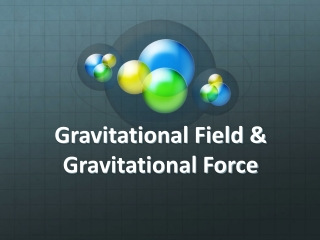DownloadDownload PresentationGravitational Field & Gravitational Force

# Gravitational Field & Gravitational Force

Download Presentation## Gravitational Field & Gravitational Force

- - - - - - - - - - - - - - - - - - - - - - - - - - - E N D - - - - - - - - - - - - - - - - - - - - - - - - - - -
##### Presentation Transcript

1. Gravitational Field & Gravitational Force

2. “Action at a distance” • The gravitational force is an attractive force between two masses. • Gravity is a field force. The masses don’t need to be in contact with each other to cause attraction.

3. Backpack, apples, ect. • Jumping with a large backpack on is a lot harder than jumping with a small backpack on. • Why? • Gravity must have something to do with the mass of the object (ie. Backpack)

4. Backpack, apples, ect. Earth, moon,… • Dropping an object on Earth is different than dropping that same object on the moon. • The object will fall to the ground in both scenarios, but the rates are different. Why? • The moon is less massive than the Earth, so falling (caused by gravity) must be directly related to the mass of one object (the Earth or the moon) causing a second object (an apple, a ball) to fall.

5. What about distance between the two masses? • It turns out, the gravitational force decreases with distance, but not linearly. • If you double the distance between two objects the force of gravity between them decreases by a factor of 4. • If you quadruple the distance between two objects, the force of gravity decreases by a factor of 16! • This is the inverse square law:

6. So what is gravity? • Gravity is the force of attraction between two masses. Where: G = Universal Gravitational Constant = 6.67x10-11 N.m2/kg2 M1 = Mass of one object (in kg) m2 = Mass of other object (in kg) r = distance between objects (measured center to center in meters)

7. Example: Determine the force of gravitational attraction between the earth (m = 5.98x1024 kg) and a 70. kg physics student flying in an airplane 40000 ft (12,000 m) above the surface of the Earth (radius of Earth = 6.3781x106m). M1 = 5.98x1024 kg m2 = 70. kg r = 12,000m + 6.3781x106m = 6.3901x106m Fgravity = 683.77 ~ 680 N

8. Field Force • Gravity is a field force, meaning the two masses will attract each other even when they aren’t in contact with each other. • This force of attraction will cause the masses to accelerate towards each other. • Masses create gravitational fields around themselves. Any other mass in that gravitational field will experience a force of attraction. • It is this gravitational field that causes gravity to be able to act at a distance on other masses.

9. Any object near the surface of the Earth will accelerate towards the Earth at 9.8 m/s2 Any object that is 3.8x108 m from the surface of the Earth will accelerate towards the Earth at 0.00272 m/s2 Earth’s Gravitational Field

10. Calculating Gravitational Fields: Where: g = gravitational field G = Universal Gravitational Constant = 6.67x10-11 N.m2/kg2 M1 = Mass of the object CREATING the field (in kg) r = distance from the center of the object creating the field to the point you are looking at. Units:

11. Calculate Earth’s Gravitational Field. MEarth = 5.98x1024 kg & radius of Earth = 6.3781x106m g = 9.80 m/s2

12. A satellite is halfway between the Earth and the moon. What is the net gravitational field at that location. MEarth = 5.98x1024 kg Mmoon = 7.36x1022 kg 1.9x108m 1.9x108m = 0.0109 N/kg 

13. What does a gravitational field of 0.0109 N/kg mean? This mass CREATES the Gravitational field This mass EXPERIENCES the Gravitational field The Gravitational field • Any object placed at that location will accelerate towards the Earth at 0.0109 m/s2 regardless of it’s mass. • It will also let us calculate the force of gravity on any object placed at that location using F = mg Gravitational field “g”

14. Back to the satellite: If a 150 kg satellite is placed in orbit halfway between the Earth and the moon, what will the force of gravity be on the satellite? g = 0.0109 N/kg Fgravity = mg Fgravity = (150kg)(0.0109N/kg) Fgravity = 1.6 N

15. The satellite is in orbit around the Earth (instead of floating off in a straight line towards Jupiter). What is the magnitude/strength of the centripetal force? This is what we will be talking about tomorrow… Fgravity = 1.6 N

16. How do the tides work?

17. What we already know: The water under the moon is closer to the moon than the center of the Earth is. So the moon’s gravity pulls harder on the water and the water “heaps up” under the moon.

18. But what about the bulge on the other side?

19. The New Part: The center of the Earth is closer to the moon than the water on the OPPOSITE side of the Earth. The moon pulls the Earth away from the water, and it appears to “heap up” too.

20. Spring Tides Neap Tides